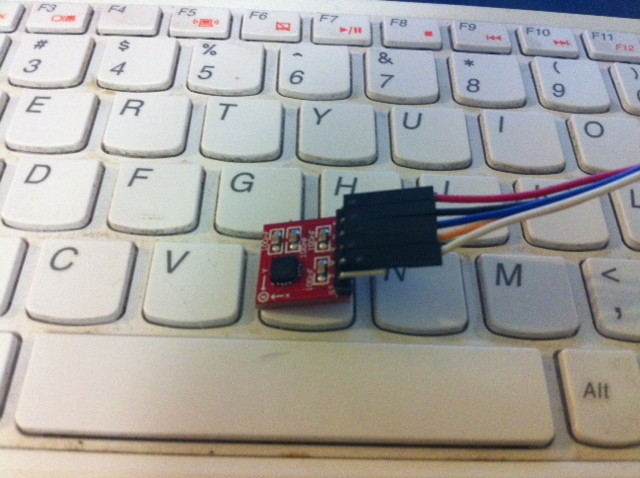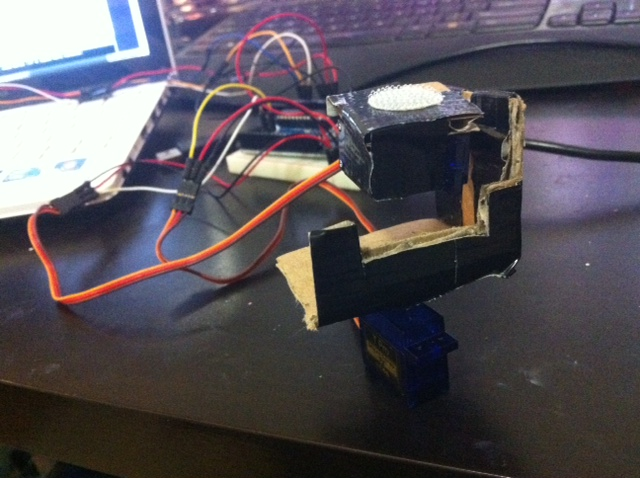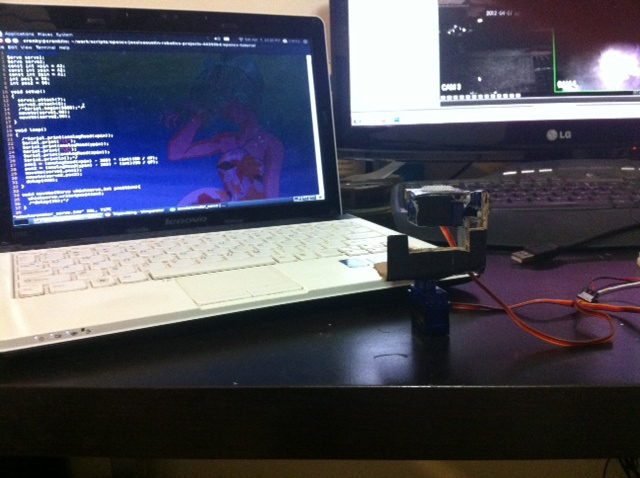I needed a break from work (real work), so I decided to spend an hour or two playing with microcontrollers again.

I’ve been itching to build a sentry gun, however, I’m still in the process of getting comfortable with opencv, face detection, pattern recognition, etc.

In the meantime, I decided this was the perfect opportunity to experiment with my 3-axis accelerometer and a couple servos.A while back, I posted an entry about my homemade Nintendo powerglove using flex sensors. This time, I want to attach an accelerometer to that same glove and use my hand to control a little missile turret.

First, I ghetto-rigged this miniature pan/tilt turret using two micro servos, cardboard, screws, and duct tape. I probably shouldn’t be using cardboard, but this is just a prototype.Next, I connected my ADXL335 3-axis accelerometer’s X,Y,Z terminals to analog pins 1-3 on my arduino. I plugged ground into ground and power into the 3.3V source (5V is too high).

I serial.printed the readings while playing with the accelerometer to find out what the range of the readings were. This is what I got:

 Min Max X 268 409 Y 263 402 Z 277 412

X was the result of tilting the accelerometer left and right.
Y was the result of tilting the accelerometer forward and back.
Z reflected the orientation of the accelerometer whether it was upright or upside down.

Now, the servos accept a digital value from 0 to 180 for a full 180 degree range.
So, how do get the arduino to convert accelerometer readings to servo outputs? Exactly the same way you derive the well-known Fahrenheit/Celsius conversion formula. In case you forgot: (skip if you already know it)

### How to Derive the Fahrenheit to Celsius Conversion Formula

You need two anchor points for each Celsius and Fahrenheit. We’ll use the water freezing and boiling temperatures.

 freezes boils C 0 100 F 32 212

Now, we represent these values in slope-intercept form:
y = mx + b
and we’ll say y is Fahrenheit.
We get 32 = 0m + b
and 212 = 100m + b
b = 32 and plugging that into the second equation, we derive that m = 9/5
Therefore, F = (9/5)*C + 32

So given the data:
268 = 0m + b
b = 268
409 = 180m + 268
141 = 180m
m = 141/180 = 47/60
Therefore: xAcc = xServo*(47/60)+268

and…
263 = b
402 = 180m + 263
134 = 180m
m = 67/90
Therefore: yAcc = yServo*(67/90) + 263

And that’s all there is to it!

Onto the code:

``````
#include <Servo.h>
Servo servo1;
Servo servo2;
const int xpin = A3;
const int ypin = A2;
const int zpin = A1;
int pos1 = 90;
int pos2 = 90;

void setup()
{
servo1.attach(7);
servo2.attach(8);
moveto(servo1,90);
moveto(servo2,90);
}

void loop()
{
pos1 = (analogRead(xpin) - 268) * (int)(60 / 47);
pos2 = (analogRead(ypin) - 263) * (int)(90 / 67);
moveto(servo1,pos1);
moveto(servo2,pos2);
delay(400);
}

void moveto(Servo whichservo,int position){
whichservo.write(position);
}
```
```Adjusting the delay value to a lesser integer makes it more responsive but I raised the delay because I naturally shake a lot and the turret looks like it’s spazzing out.

So what is next? I need to mount a little water pistol and detect humans using the openCV library. If and when I do that, I will do a follow-up.

From → Hacks

One Comment